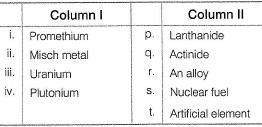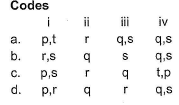Courses

# Test: f-Block Elements (Lanthanides)- I

## 25 Questions MCQ Test Chemistry for JEE | Test: f-Block Elements (Lanthanides)- I

Description
This mock test of Test: f-Block Elements (Lanthanides)- I for JEE helps you for every JEE entrance exam. This contains 25 Multiple Choice Questions for JEE Test: f-Block Elements (Lanthanides)- I (mcq) to study with solutions a complete question bank. The solved questions answers in this Test: f-Block Elements (Lanthanides)- I quiz give you a good mix of easy questions and tough questions. JEE students definitely take this Test: f-Block Elements (Lanthanides)- I exercise for a better result in the exam. You can find other Test: f-Block Elements (Lanthanides)- I extra questions, long questions & short questions for JEE on EduRev as well by searching above.
QUESTION: 1

### Only One Option Correct Type Direction (Q. Nos. 1- 10) This section contains 10 multiple choice questions. Each question has four choices (a), (b), (c) and (d), out of which ONLY ONE is correct. Q.  Set of continuous atomic numbers of elements are present in the same group as well as same period.

Solution:

Er (68), Tm (69), Yb (70) and Lu (71) elements are present in same group as well as same period.

QUESTION: 2

### The element with atomic number 62 belongs

Solution:

Samarium (Sm) (Z = 62) belongs to III B group and 6th period.

QUESTION: 3

### The radius of lanthanum is 1.06 Å. Then which of the following is closest to the radius of Lu3+ ion

Solution:

Due to lanthanoid contraction.

QUESTION: 4

+ 4 ion of which has half-filled 4f subshell

Solution:

Tb (Z = 65) have [Xe] 4f95d06s2 configuration. Tb4+ have half-filled 4f subshell, i.e. 4f7.

QUESTION: 5

Lanthanide for which + II and + III oxidation states are common is

Solution:

Europium (Eu) has [Xe] 4f75d06s2 configuration. The oxidation state of + 2 and + 3 are exhibited by these elements, e.g. Eu2+ is[Xe] 4f7and Eu3+ is [Xe]4f6.

QUESTION: 6

An alloy of lanthanide

Solution:

Misch metal is lanthanide metal (95%) and iron (5%) and traces of S, C, Ca, Al.

QUESTION: 7

Across the lanthanide series, the basicity of the lanthanide hydroxides

Solution:

The basicity decreases from Ce(OH)3 to Lu(OH)3. Due to lanthanide contraction, the decrease in the size of the cation increases the covalent character between the lanthanide ion and hydroxide ion thereby, reducing the basic character of the lanthanide hydroxide.

QUESTION: 8

Gadolinium belongs to 4f series. Its atomic number is 64. Which of the following is the correct electronic configuration of gadolinium?

Solution:

Gadolinium (Gd) have [Xe] 4f75d16s2 configuration.

QUESTION: 9

Lanthanides contraction causes

Solution:

Electronegativity increases as we move from left to right due to lanthanide contraction.

QUESTION: 10

Which one of the following statement(s) is/are incorrect for lanthanides?

Solution:

The hardness increases with increasing atomic number.

*Multiple options can be correct
QUESTION: 11

One or More than One Options Correct Type

Direction (Q. Nos. 11-15) This section contains 5 multiple choice questions. Each question has four choices (a), (b), (c) and (d), out of which ONE or MORE THAN ONE are correct.

Q.

Which of the following statements are incorrect regarding lanthanides?

Solution:

Ce3+ (4f1) and Yb3+ (f13) are colourless because they do not absorb in the visible region. The E° value for Ce4+ /Ce3+ is + 1.74 V which suggest it is strong oxidising agent.

QUESTION: 12

Observe the following statements. Which is correct statement regarding lanthanides?

Solution:

The correct answer is option B
Ce shows +4 oxidation state in aq.solution
Ce (58) to lu (71)-  lanthanide
Th (90) to Lr (103)- actinoids.

*Multiple options can be correct
QUESTION: 13

The colourless ions among the lanthanides are

Solution:

La3+, Gd3+ and Lu3+ have empty (4f0) half-filled (4f7) and full-filled (4f14) orbitals. Ce3+ (4f1) is colourless because it does not absorb in the visible region.

*Multiple options can be correct
QUESTION: 14

The correct statements among the following are

Solution:

Basic strength decreases from La(OH)3 to Lu (OH)3,

*Multiple options can be correct
QUESTION: 15

Which of the following pairs have same atomic size?

Solution:

The pair of elements Zr - Hf, Nb - Ta, Mo - W possess almost the same size. After the lanthanoids the increase in radii from second to third transition series almost vanishes.

QUESTION: 16

Comprehension Type

Direction (Q. Nos. 16 and 17) This section contains a paragraph, describing theory, experiments, data, etc. Two questions related to the paragraph have been given. Each question has only one correct answer among the four given options (a), (b), (c) and (d).

Passage

The observed oxidation state of lanthanides either in solution or in insoluble compounds form tripositive cations. Based on the ionisation enthalpies and hydration energy factors, it is concluded that tripositive species is more stable than di or tetrapositive species in aqueous solution. In general, ions having half-filled (Tb4+ , Eu2+ , Gd3+) or completely filled 4f orbitals (Yb2+, Lu3+) or noble gas configuration (La3+, Ce4+) are stable. This is supported by their SRP values. Ions with + 4 oxidation state act as oxidising and ions having + 2 oxidation state act as reducing agents. Ions having same number of unpaired electrons in f-orbitals possess same colour.

Q.

Observe the following equations.
Sm3+(aq) + e- → Sm2+ (aq), E° = - 1.55 V
Eu3+(aq) + e- → Eu2+ (aq), E° = - 0.43 V
Yb3+(aq) + e- → Yb2+ (aq), E° = - 1.55V

Based on the above equations, the correct reducing strength is in the order of

Solution:

More negative the SRP value, stronger is the reducing agent.

QUESTION: 17

The observed oxidation state of lanthanides either in solution or in insoluble compounds form tripositive cations. Based on the ionisation enthalpies and hydration energy factors, it is concluded that tripositive species is more stable than di or tetrapositive species in aqueous solution. In general, ions having half-filled (Tb4+ , Eu2+ , Gd3+) or completely filled 4f orbitals (Yb2+, Lu3+) or noble gas configuration (La3+, Ce4+) are stable. This is supported by their SRP values. Ions with + 4 oxidation state act as oxidising and ions having + 2 oxidation state act as reducing agents. Ions having same number of unpaired electrons in f-orbitals possess same colour.

Q.

Which is correct statement?

Solution:

Ce4+ is [Xe] 4f0, it tends to revert to more stable oxidation state of +3 by gain of an electron. That is why, in aqueous solution, Ce4+ is good oxidising agent.

QUESTION: 18

Matching List Type

Direction (Q. No. 18) Choices for the correct combination of elements from Column I and Column II are given as options (a), (b), (c) and (d), out of which one is correct.

Q.

Match the Column I with Column II and mark the correct option from the codes given below.Solution:

(i) → (p.t) (ii) → (r) (iii) → (q,s) (iv) → (q,s)

*Answer can only contain numeric values
QUESTION: 19

One Integer Value Correct Type

Direction (Q. Nos. 19-23) This section contains 5 questions. When worked out will result in an integer from 0 to 9 (both inclusive).

Q.

Number of species that have half-filled 4f subshell among Gd, Lu, Eu, Yb, Eu2+ Gd3+ ,Eu3+ ,Ce4+ ,Tb4+.

Solution:

Gd: [Xe] 4f75d16s2,
Lu: [Xe] 4f145d16s2,
Eu: [Xe] 4f75d06s2,
Yb: [Xe] 4f145d06s2,
Eu2+ : [Xe] 4f75d06s0,
Gd3+ : [Xe] 4f75d06s0,
Eu3+ : [Xe] 4f65d06s0,
Tb4+: [Xe] 4f75d06s0,
Ce4+: [Xe] 4f05d06s0,

*Answer can only contain numeric values
QUESTION: 20

Number of unpaired electrons in ytterbium is/are

Solution:

Ytterbium has configuration [Xe] 4f145d06s2

*Answer can only contain numeric values
QUESTION: 21

Number of diamagnetic lanthanide ions among

La3+, Ce3+ Ce4+, Gd3+,Yb2+,Yb3+,Lu2+, Lu3+ are

Solution:

La3+, Ce4+, Yb2+ and Lu3+ are diamagnetic in nature.

*Answer can only contain numeric values
QUESTION: 22

Number of good reducing agents among

Ce4+, Eu2+, Sm2+,Yb2+,Tb4+,La3+ Ce3+ are

Solution:

Eu2+ ,Sm2+ ,Yb2+ are reducing agents.

*Answer can only contain numeric values
QUESTION: 23

The highest oxidation state shown by any transition element is

Solution:

The highest oxidation state shown by the transition element is Ru (+8) in 4d series and Os in 5d series.

QUESTION: 24

Statement Type

Direction (Q. Nos. 24 and 25) This section is based on Statement I and Statement II. Select the correct answer from the codes given below.

Q.

Statement I : The second and third rows of transition elements resemble each other much more than they resemble the first row.

Statement II : Due to lanthanide contraction, the atomic radii of the second and the third row transition elements.

Solution:

Both statements I and II are correct. Statement II correctly explains the statement I.

QUESTION: 25

Statement I : Many trivalent lanthanide ions are coloured both in solid state and in aqueous solution.

Statement II : Colour of these ions is due to the presence of f-electrons.

Solution:

Many trivalent lanthanide ions are coloured due to the presence of f-electrons, in solid state as well as in aqueous solutions.